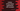# Python string lstrip() method explanation with example## Python string lstrip() method explanation with example:

Python strings have one method called lstrip that can be used to delete leading whitespaces or any other leading characters from a string. This is a useful method and we can use this method to quickly remove the leading characters or whitespaces from a string in Python.

In this post, we will learn how to use lstrip method with examples.

### Definition of lstrip:

lstrip is defined as like below:

``str.lstrip([chars])``

Here,

• We are calling lstrip on the string str, which is the original string.
• chars is an optional value. This is a string or this is the set of characters that we want to remove from the string. As this is an optional value, if we provide None or if we don’t provide any value, then it will remove whitespaces from the start of the string.

It will remove all combinations of the characters of chars. It is not the prefix of the string that we are removing.

#### Return value of lstrip:

This method will return the newly created string. It will return a copy of the original string.

Let’s try lstrip with different types of parameters.

### Example 1: lstrip without any parameters:

Let’s try lstrip without any parameters first:

``````str_1 = '       hello    '
str_2 = '\t   \t   hello   '
str_3 = '\n\t\thello'
str_4 = ''

print(f'str_1 {[str_1]}')
print(f'str_2 {[str_2]}')
print(f'str_3 {[str_3]}')
print(f'str_4 {[str_4]}')

print(f'\nwith lstrip: \n')

print(f'str_1 {[str_1.lstrip()]}')
print(f'str_2 {[str_2.lstrip()]}')
print(f'str_3 {[str_3.lstrip()]}')
print(f'str_4 {[str_4.lstrip()]}')``````

It will print:

``````str_1 ['       hello    ']
str_2 ['\t   \t   hello   ']
str_3 ['\n\t\thello']
str_4 ['']

with lstrip:

str_1 ['hello    ']
str_2 ['hello   ']
str_3 ['hello']
str_4 ['']``````

All strings are wrapped with square brackets so that the strings are easily distinguishable.

As you can see here, the leftmost whitespaces are removed from the strings.

### Example 2: lstrip with characters:

Let’s use lstrip with characters as the parameters. We can pass a string to the lstrip method and it will remove all the set of characters from the beginning of the string.

Let’s write down the program:

``````str_1 = '12345hello    '
str_2 = 'aeioauaahello   '
str_3 = '-----hello'
str_4 = '----yyyhello'

print(f'str_1 {[str_1]}')
print(f'str_2 {[str_2]}')
print(f'str_3 {[str_3]}')
print(f'str_4 {[str_4]}')

print(f'\nwith lstrip: \n')

print(f"str_1 {[str_1.lstrip('54321')]}")
print(f"str_2 {[str_2.lstrip('aeiou')]}")
print(f"str_3 {[str_3.lstrip('-')]}")
print(f"str_4 {[str_4.lstrip('y-')]}")``````

It will print:

``````str_1 ['12345hello    ']
str_2 ['aeioauaahello   ']
str_3 ['-----hello']
str_4 ['----yyyhello']

with lstrip:

str_1 ['hello    ']
str_2 ['hello   ']
str_3 ['hello']
str_4 ['hello']``````

You don’t have to provide the exact string that you want to remove. You need to provide a string and any of the character from that string will be removed.

### Using lstrip to remove the first n characters from a string:

We can’t use lstrip to remove the first n characters from a string. Because, it will use the combinations of the characters and those characters will be removed.

So, let’s check the below program:

``````msg = '1023420 Hours'

print(msg.lstrip('10234'))``````

We want to remove ‘10234’ from the string msg. But, if we pass 10234 to lstrip, it will remove all the digits.

`` Hours``

So, we can’t use lstrip to remove a leading substring from a string.